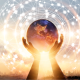# IF WE ARE ALL THAT IS: February 25-27, 2022

If we are all that is,
the kingdom of absolute good,
infinite miracles expressing as us . . .
how would we dance?

How would we talk?
How would we grow?
How would we spin?
How would we imagine?
How would we know?

If we are the grace of love
that never stops giving,
how would we rise?
How would we walk?
How would we change?
How would we evolve?

How would we trust?
How would we create?

If we were the kingdom of the universe,
how would we heal?
How would we plant?
How owuld we drive?
How would we swim?

How would we eat?
How would we give?

If we are all that is,
a oneness of infinite good,
how would open our eyes
to see ourselves
for the very first time?

1.Paulette Lucas says:
1.#lovingfactor says: